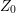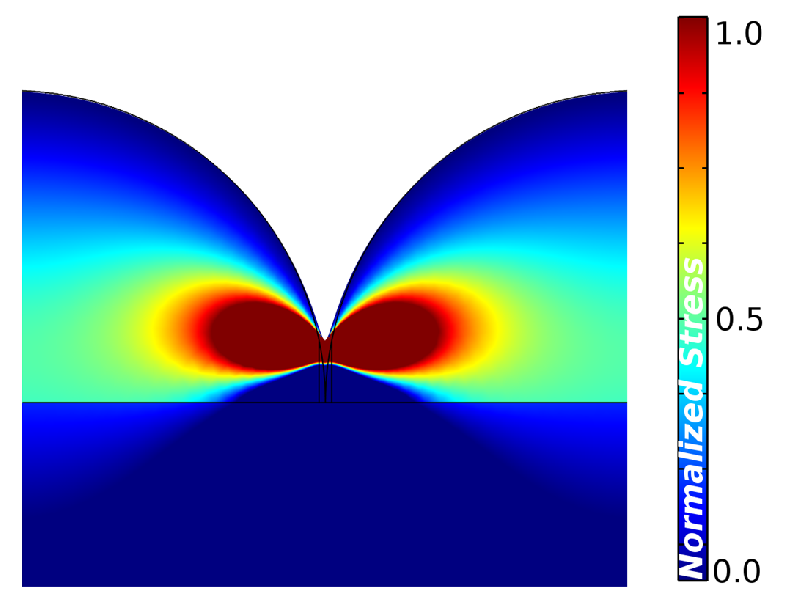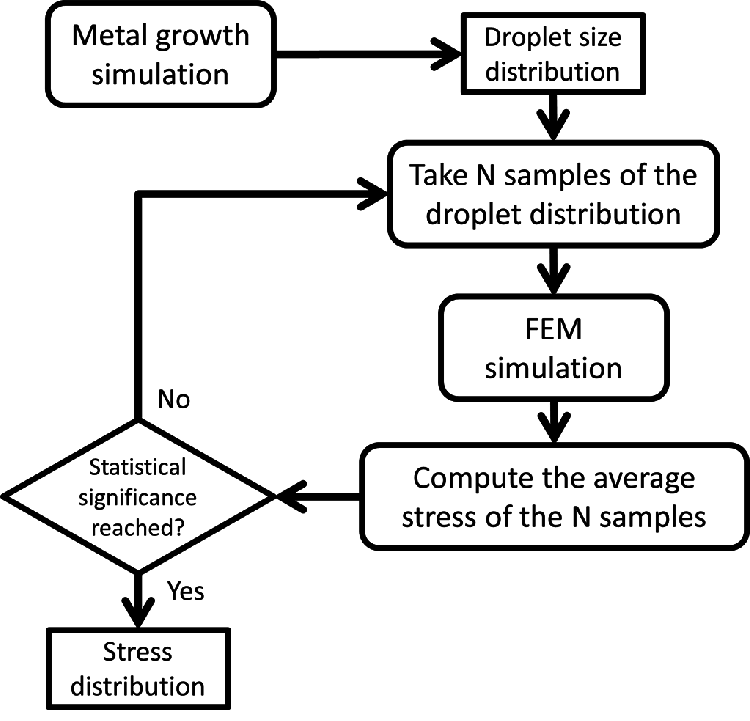#### 6.5.2Coalescence Simulation

After a VW growth simulation, a sample is taken from the droplet size distribution and a FEM simulation is performed in order to compute the stress. The setup follows Seel’s scheme, butis not computed from the FEM simulations; instead the numerical minimization of (6.8) regardingis used. This approach is faster, because it avoids extra FEM simulations in the search for. A FEM simulation example of a grain encounter is shown in Fig. 6.11.Figure 6.11.: FEM simulation of a single encounter between two droplets. Symmetry conditions are employed from both sides. A prescribed displacement is applied at the surface of both grains up to a critical height.

The calculated stresses are then averaged and the entire process is repeated for a new sample from the droplets’ size distribution, until it reaches statistical significance (usually in the order of several thousand simulations). Finally, an estimate for the average stress in the film can be calculated together with the confidence interval. The method is summarized in Fig. 6.12.Figure 6.12.: Method to estimate the average residual stress.